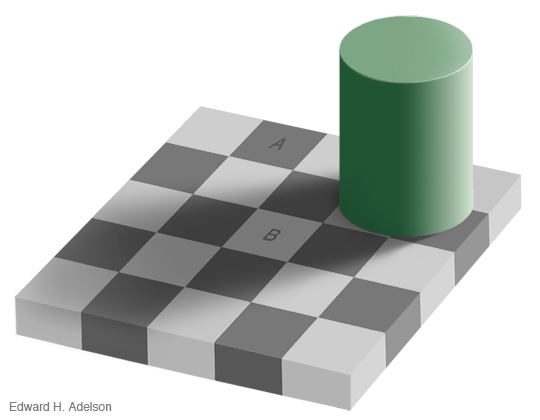# Can you explain the missing square?

Carefully observe these two triangles of equal size: the forms were merely rearranged. A square is missing in the second configuration! What is the explanation?*

Another optical illusion: Squares A and B are the same shade of gray.
Don’t believe it? Go here for the explanation.* And here’s the explanation for the missing square.

Posted in Ur

## 2 Replies to “Can you explain the missing square?”

1.Tony Reix says:

Easy. But I miss the words in English.
Let’s try:
– the ratio of Red triangle is 3/8 .
– the ratio of Green triangle is 2/5 .
– the ratio of the whole trianle should be 5/13 .
3 different ratios.
So, the surface of top triangle is: 3*8/2 + 2*5/2 + 3*5 = 32 though the surface of down triangle is: 3*8/2 + 2*5/2 + 2*8 = 33. Difference is 1 square.
Tony

2.Andy Southern says:

Just say that as the two triangles are different gradiants, one arrangement is concave and one arrangement is convex.

The hole appears in the one which is convex.

I spotted this by eye, but it’s easier to see by holding a ruler or straight edge against the screen.

The two arrangements are deviant from the apparent ideal by 1/2 a unit square.

This site uses Akismet to reduce spam. Learn how your comment data is processed.